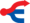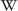# Bit (digit)The title of this article is ambiguous. Bit (Disambiguation).

A bit is the smallest unit of data storage in computer science. The term is short for Binary Digit.

A single bit must be in one of two states. These two states are most often thought of as one (1) and zero (0). But they can just as correctly be thought of as "on" and "off", or "true" and "false". Multiple bits, when combined together, can be interpreted as larger numbers, or as pictures (bitmaps) or as typographical characters. For instance, the bit-combination 01000001 in the Commodore 64 is the decimal value 65 or the character value A.

In modern computers, such as the Commodore 64, eight bits are bundled together as one byte. The first, or least-significant bit is viewed right-most and named bit zero (0). The last, or most-significant bit, is viewed left-most and named bit seven (7). For instance, the eight-bit word 00000001 is one (1) in decimal, but the eight-bit word 10000000 is one hundred twenty-eight (128) in decimal. Similarly 00000010 is two (2) and 00000100 is four (4), and so on.

A computer's word-size is designated in bits. An eight-bit computer, like the Commodore 64, has an eight-bit word size. A sixteen-bit computer, such as the IBM PC, has a sixteen-bit word size.

Data transfer rates are typically given in bits per second (b/S, or BPS). The symbol for a bit is b, as in b/S.

Also n bits can be combined to new units:

``` 2 bits: crumb, quad, quarter, tayste, tydbit
3 bits: basic of octalnumbersystems
4 bits: half-byte, nibble, basic of hexadecimalsystem
5 bits: nickle
8 bits: byte, today standard-unit
10 bits: deckle
16 bits: playte, chawmp (used on 32-bit) or in "low- and high-Byte" (used on 8-bit)
18 bits: chawmp (uesed on 36-bit)
32 bits: dynner, gawble (used on 32-bit)
48 bits: gawble
```Wikipedia: Bit